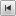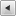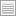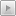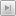# Print of mail alias tree

print-alias-tree.php
```#!/usr/local/bin/php
<?php

// \$Id: a2tree.php,v 1.1 2016/11/02 12:18:48 root Exp root \$

function print_alias (\$con, \$address, \$level) {

if (\$level > 7) {
echo str_repeat("|\t", \$level)." !!!!! too many levels, more 7 !!!\n";
return;
}

\$table = pg_query(\$con, \$query);
if(pg_num_rows(\$table) == 0) {
\$query = "select distinct username from mailbox where username = '\$address' and active = 'true' limit 1;";
\$table_user = pg_query(\$con, \$query);
if(pg_num_rows(\$table_user) == 0) {
} else {
}
pg_free_result(\$table_user);
} else {
\$row = pg_fetch_assoc(\$table);
\$goto = \$row['goto'];

\$rcpt = strtok(\$goto, ",");
while (\$rcpt !== false) {
print_alias(\$con, \$rcpt, \$level+1);
\$rcpt = strtok(",");
}
}
pg_free_result(\$table);
}

function _main (\$mx) {
echo "--- ".\$mx." -------------------------------------------------------------------\n";
\$con = pg_pconnect("host=\$mx port=5432 dbname=mail user=xxxxxx password=zzzzz");
printf("Connected successfully\n");

\$query = "select distinct address from alias where active = 'true' order by address;";

\$table = pg_query(\$con, \$query);
\$count_rows = pg_num_rows(\$table);
printf("count rows = %d\n", \$count_rows);

while (\$row = pg_fetch_assoc(\$table)) {
}

pg_free_result(\$table);
pg_close(\$con);
}

_main("mx");

//EOF```